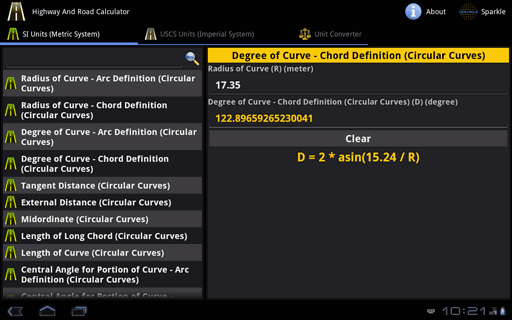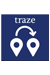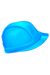Highway + Road Calculator for Android TabletSummary:
69 Calculators and Converters related to Highway Engineering and Roads. Available in both Metric and Imperial Units with Formulas. Automatic Calculations and Accurate Conversions.
2.0
Android
Category:
Education,Calculator
\$2.99
Requiements:
Works with all Android Tablet
Overview

Highway and Road Calculator contains 69 Calculators and Converters, that can quickly and easily calculate and convert different Highway, Road and Civil Engineering parameters. Automatic & Accurate Calculations and Conversions with every Unit and Value Changes. Available in Imperial and Metric Units. Most Comprehensive Highway and Road Calculator.Available in Metric and Imperial Units

Highway and Road Calculator contains following 37 Calculators:

• Radius of Curve - Arc Definition (Circular Curves)
• Radius of Curve - Chord Definition (Circular Curves)
• Degree of Curve - Arc Definition (Circular Curves)
• Degree of Curve - Chord Definition (Circular Curves)
• Tangent Distance (Circular Curves)
• External Distance (Circular Curves)
• Midordinate (Circular Curves)
• Length of Long Chord (Circular Curves)
• Length of Curve (Circular Curves)
• Central Angle for Portion of Curve - Arc Definition (Circular Curves)
• Central Angle for Portion of Curve - Chord Definition (Circular Curves)
• Tangent Offset (Circular Curves)
• Chord Offset (Circular Curves)

• Rate of Change of Grade (Parabolic Curves)
• Elevation of Point of Vertical Curvature (Parabolic Curves)
• Elevation of Point x distant from Point of Vertical Curvature (Parabolic Curves)
• Distance from Point of Vertical Curvature to Lowest Point on a Sag Curve/Highest Point on a Summit Curve (Parabolic Curves)
• Elevation of Lowest Point on a Sag Curve/Highest Point on a Summit Curve (Parabolic Curves)

• Minimum Length of Crest Vertical Curves (Sight Distance < Length of Vertical Curves)
• Minimum Length of Crest Vertical Curves (Sight Distance > Length of Vertical Curves)
• Rate of Vertical Curvature

• Structural Number (Surface Course)
• Structural Number (Base Course)
• Structural Number (Subbase Course)
• Structural Number (Pavements)

• Minimum Length of Spiral Curve
• Thrust of Structure (Culverts)
• Flexibility Factor
• Ring-Compression Stress

• Horizontal Deflection of Pipe (Iowa Formula)
• Design Pressure - Corrugated Steel Structures (Height of Cover < Pipe Diameter)
• Design Pressure - Corrugated Steel Structures (Height of Cover >= Pipe Diameter)
• Compressive Thrust (Conduit Walls)
• Ultimate Wall Stress (294 > Ratio of Pipe Diameter and Radius of Gyration of Pipe Cross Section < 500)
• Ultimate Wall Stress (Ratio of Pipe Diameter and Radius of Gyration of Pipe Cross Section > 500)
• Design Stress of Wall
• Pipe Wall Area
Highway and Road Calculator contains following 32 Converters:

• Acceleration
• Angle
• Area
• Density
• Energy/Work
• Flow Rate (Mass)
• Flow Rate (Volume)
• Fluid
• Force
• Frequency
• Hardness
• Length
• Mass
• Metric Weight
• Metrology
• Moment of Force
• Moment of Inertia
• Prefixes
• Pressure
• Specific Heat Capacity
• Specific Volume
• Temperature
• Thermal Conductivity
• Thermal Expansion
• Time
• Torque
• Velocity
• Viscosity (Dynamic)
• Viscosity (Oil & Water)
• Viscosity (Kinematic)
• Volume
Key Features:
• Complete coverage of calculators and converters in Highway, Road and Civil Engineering Parameters.
• Automatic Calculation & Conversion of the Output with respect to changes in the Input/Options/Units.
• Formulas are provided for each calculator.
• Values of Higher Order can also be calculated.
• Extremely Accurate Calculations and Conversions.
• Professionally and Newly designed user-interface that speeds up Data Entry, Easy Viewing and Calculation Speed.
Most Comprehensive Highway and Road Calculator

•  Traze - reach ur friendsShare location with friends & view theirs. Navigate & make calls, all in one go. Traze enables two-way communication. Its never been easier to reach someone. More...Civil Engineering Pack for Android Tablet574 Calculators & Converters related to Civil, Beams, Columns, Piling, Concrete, Survey, Soil & Earthwork, Structural Engineering, Bridges, Highway & Road, Hydraulics and Timber. More...